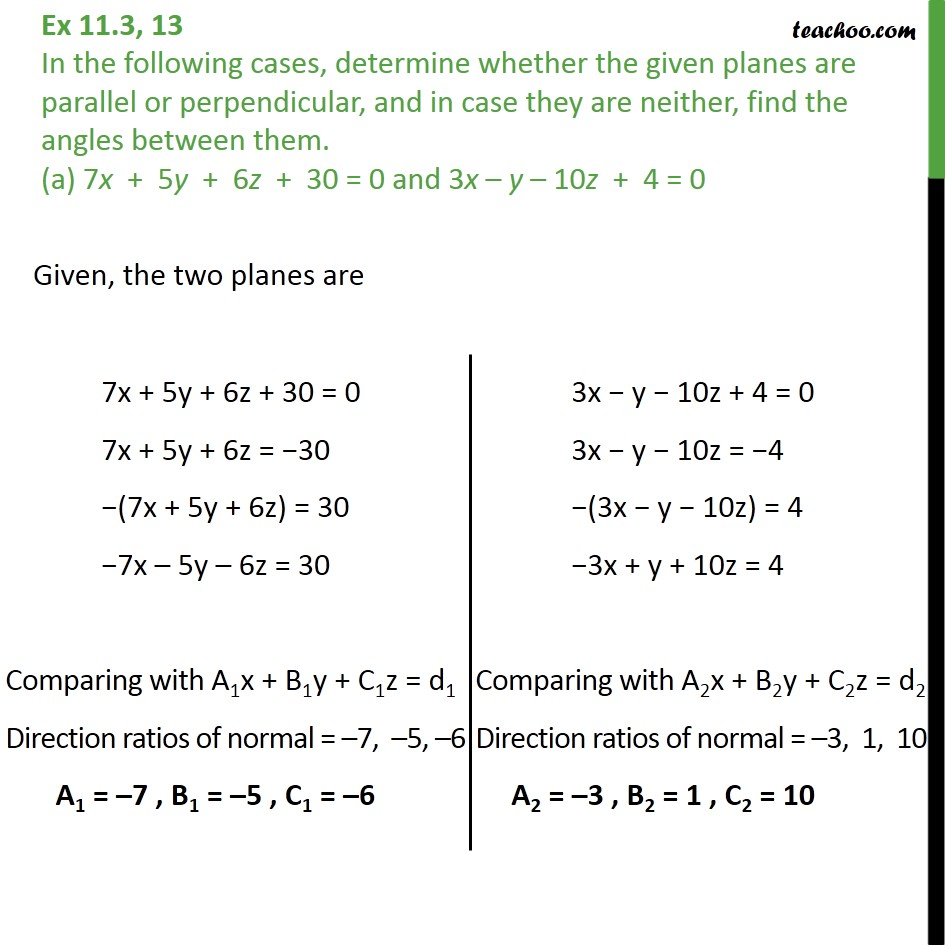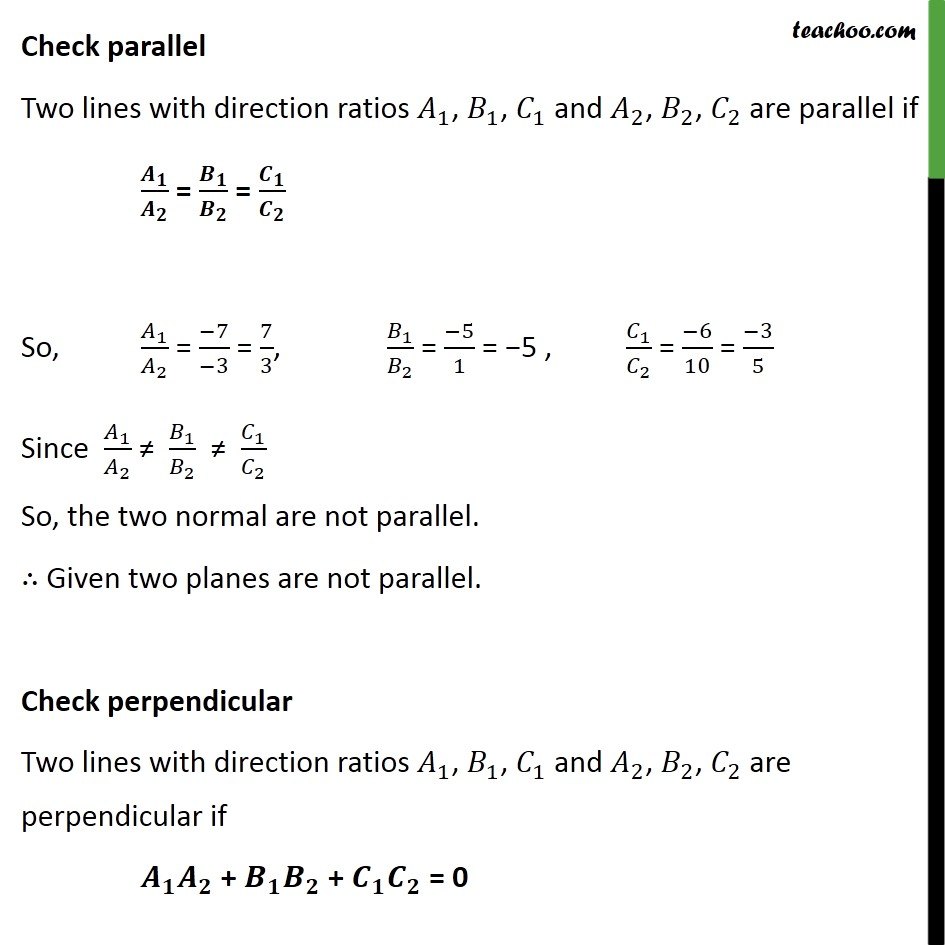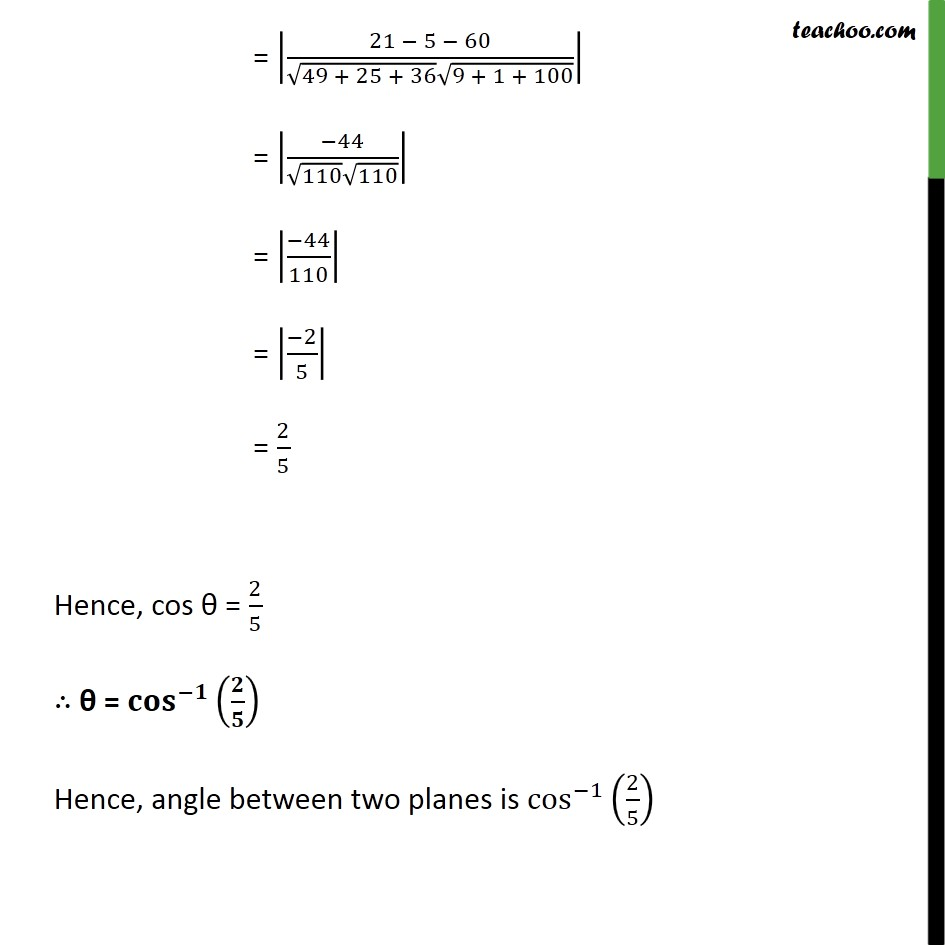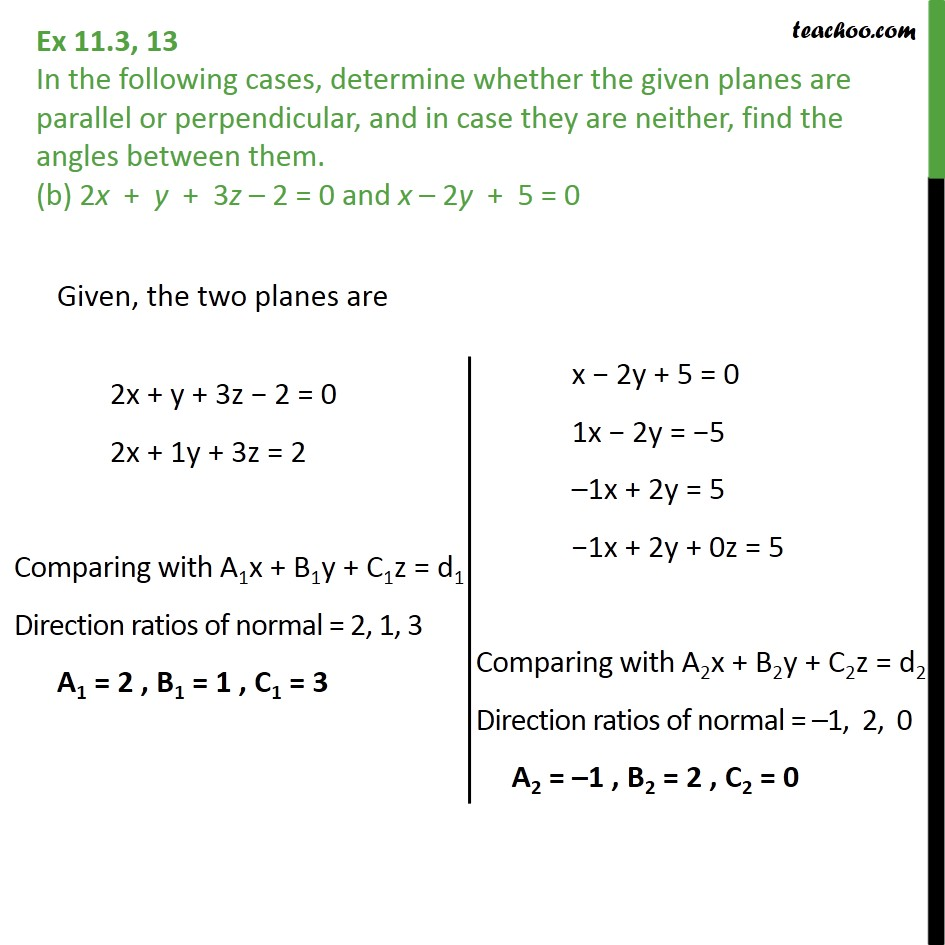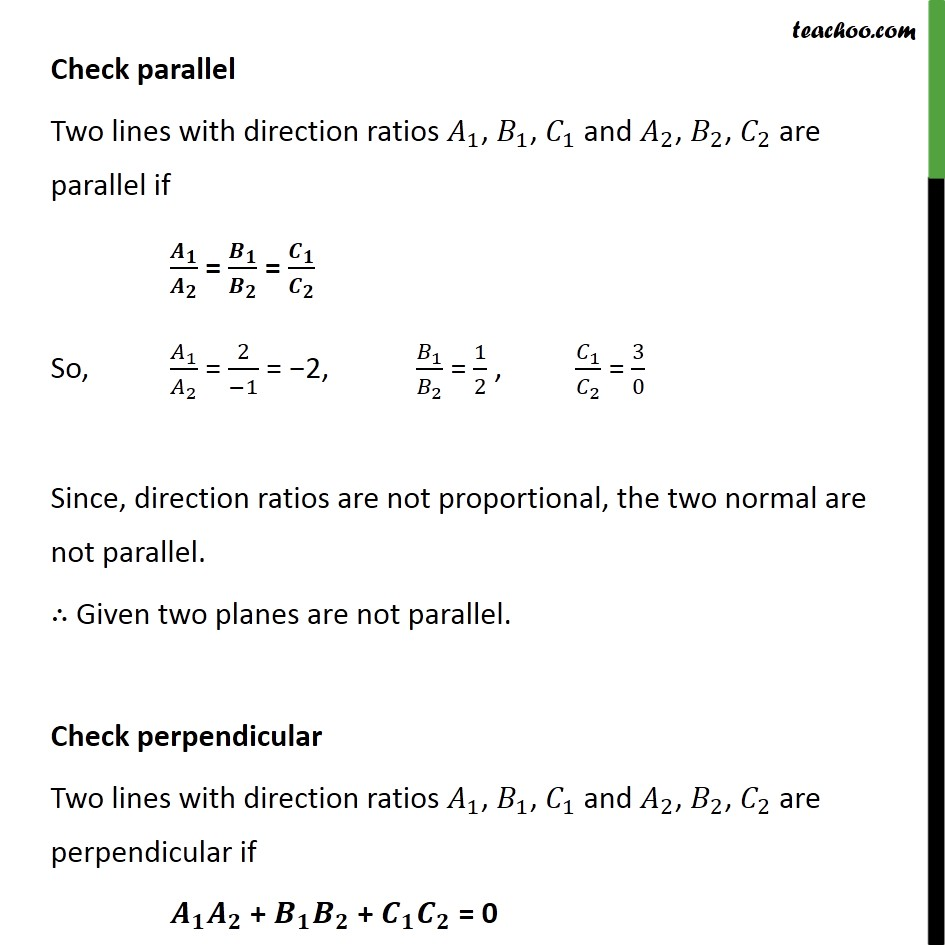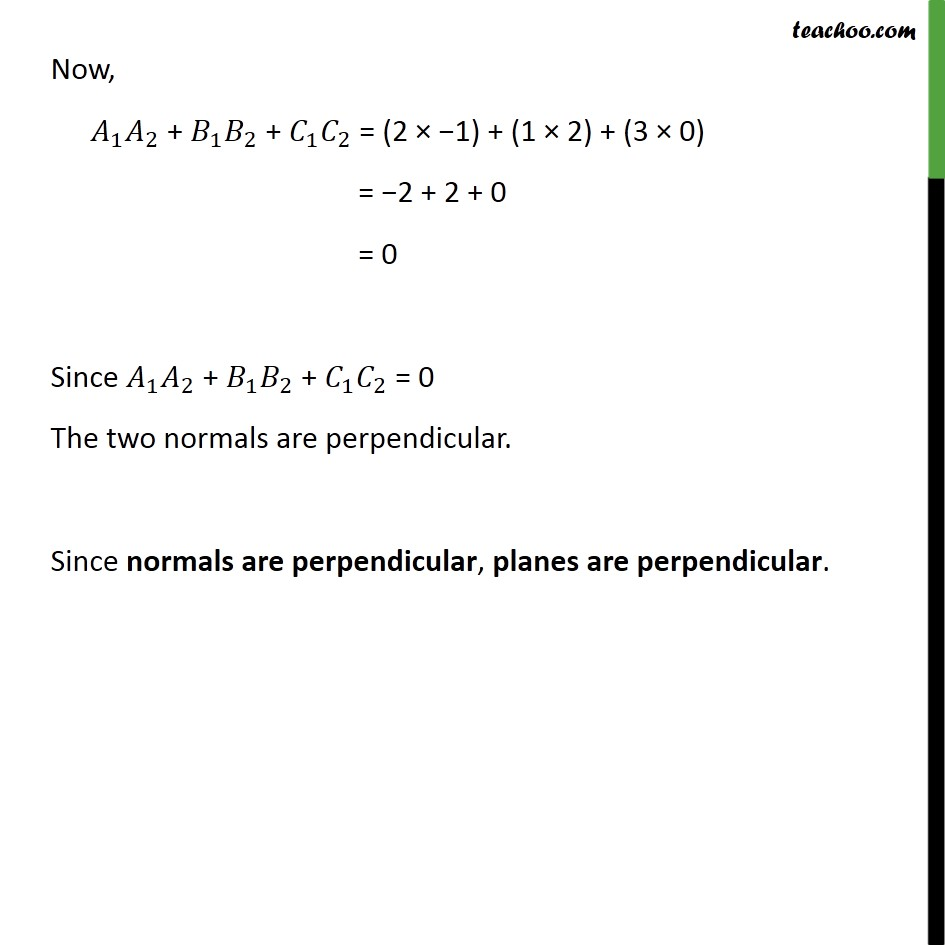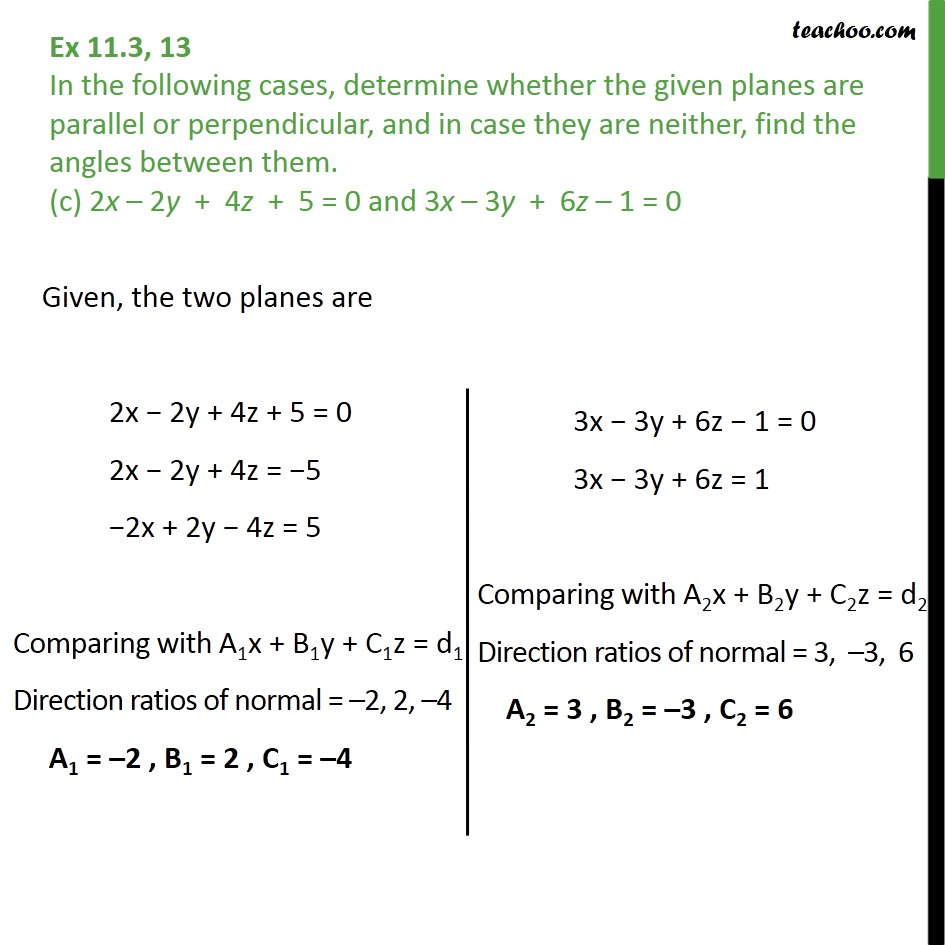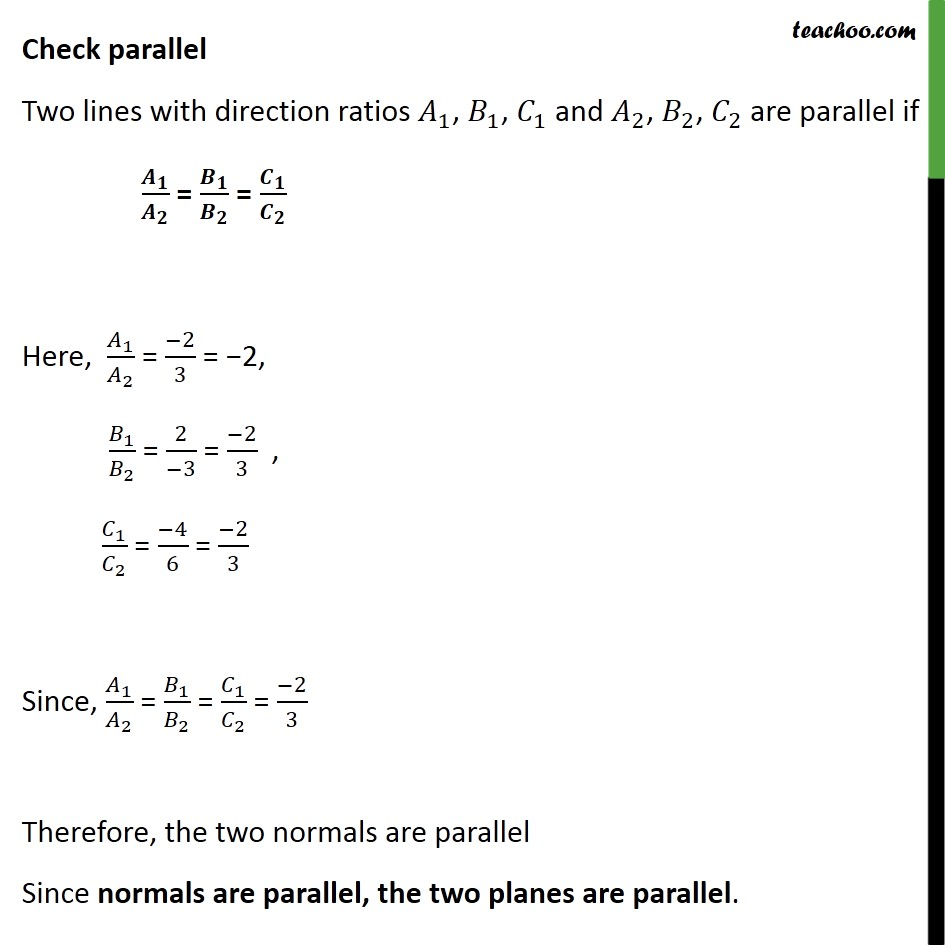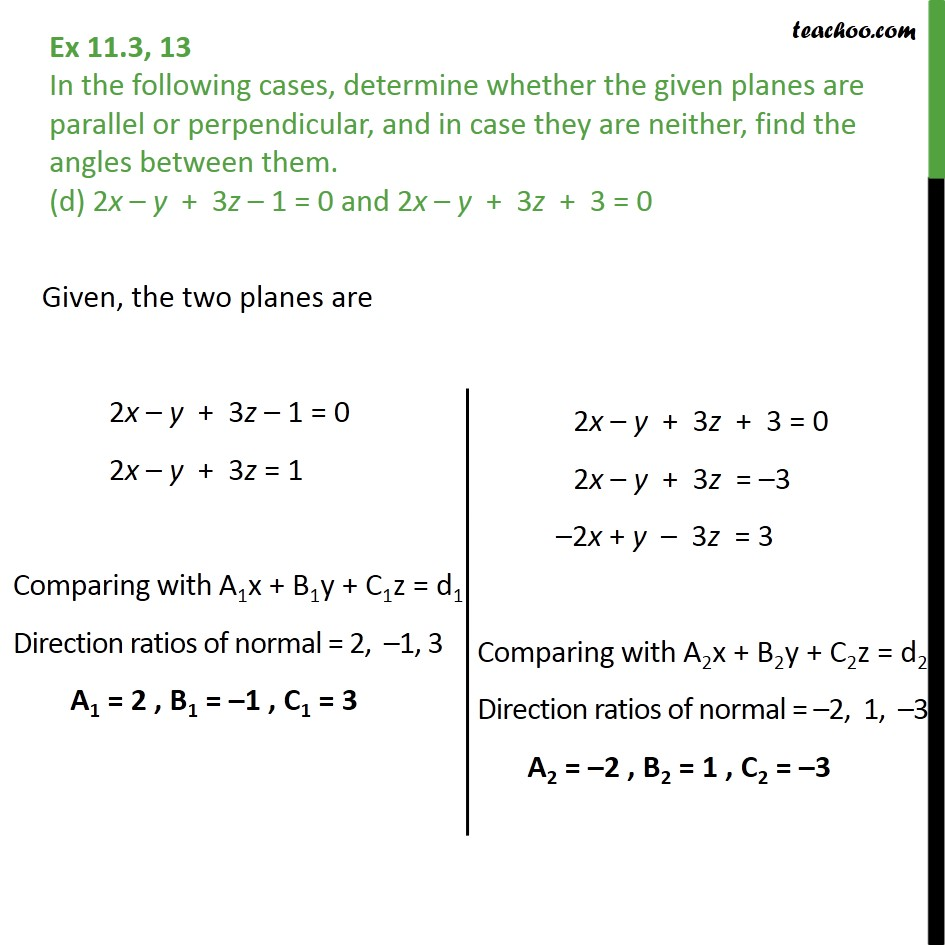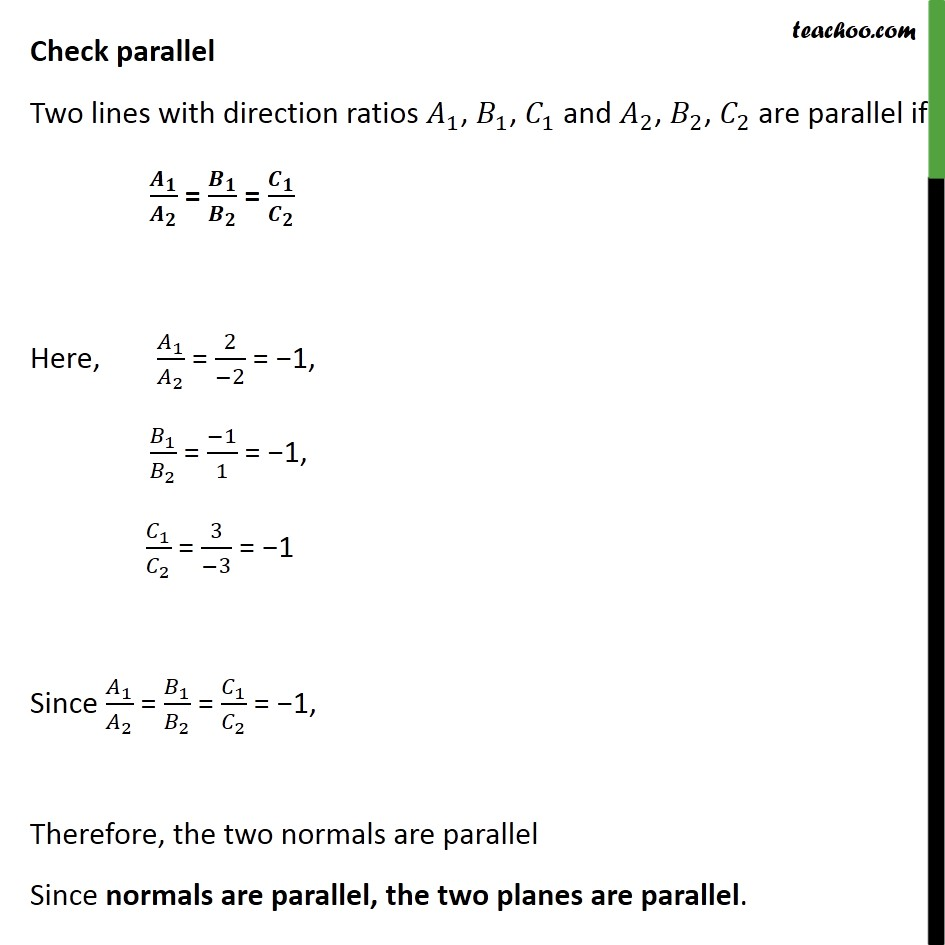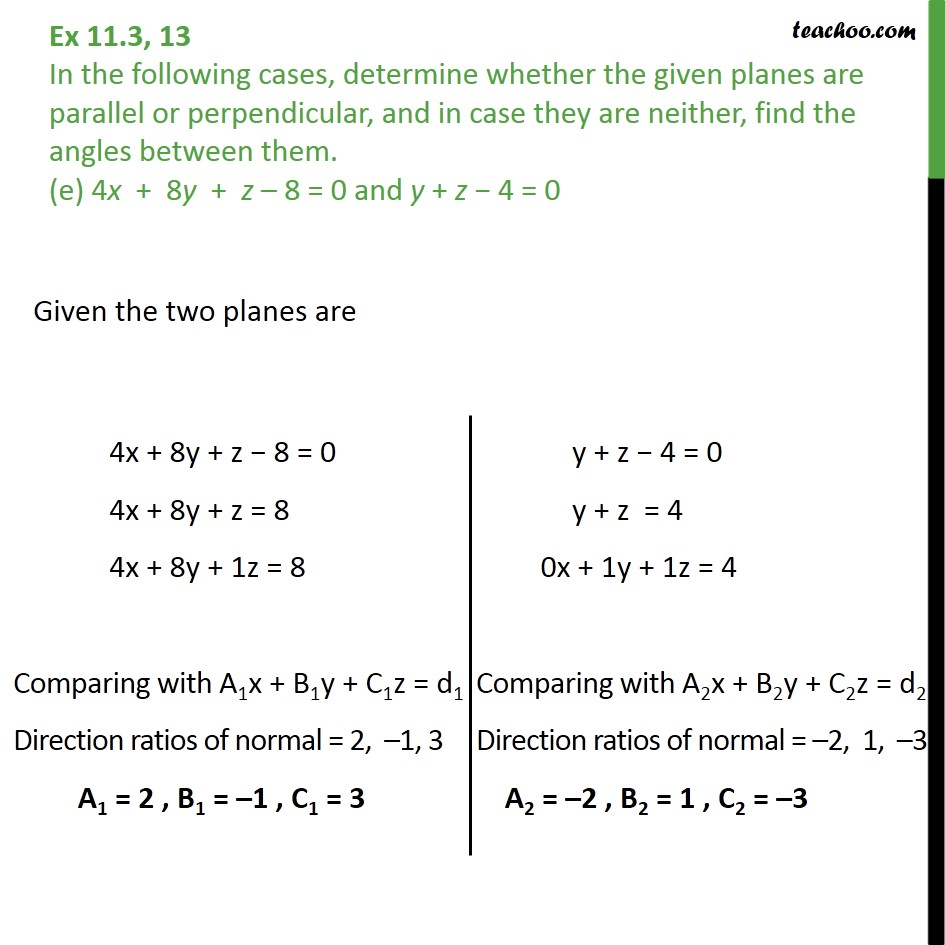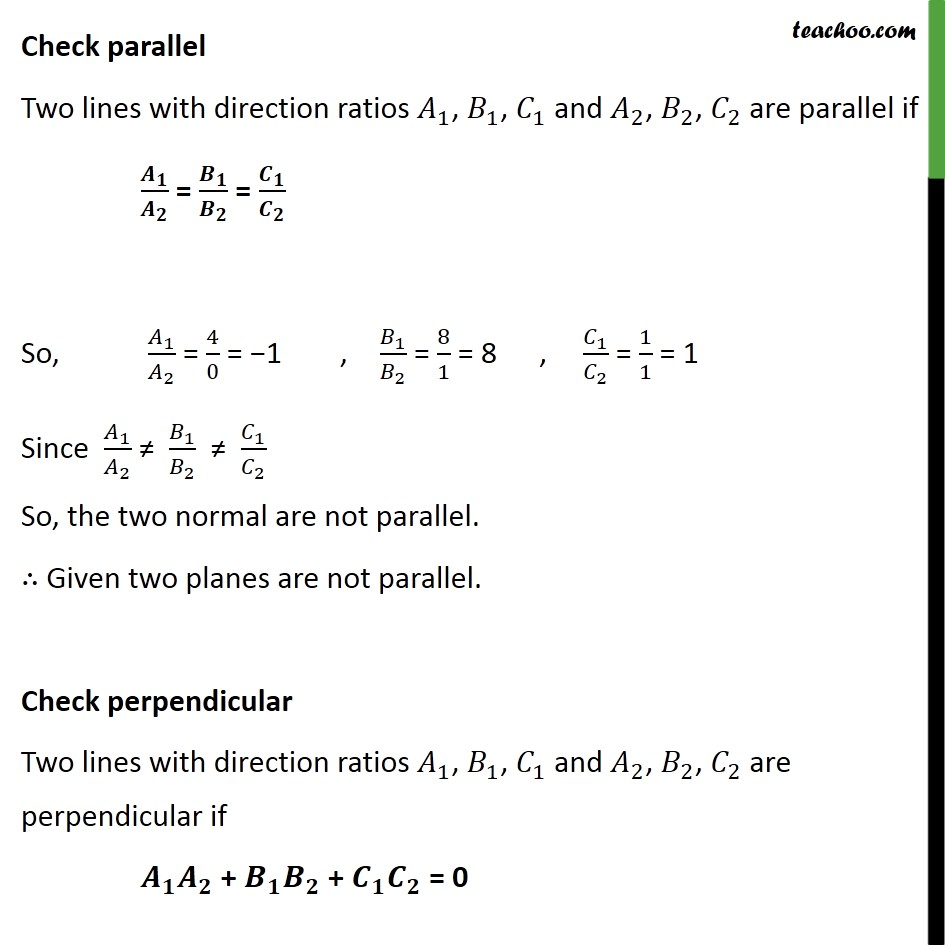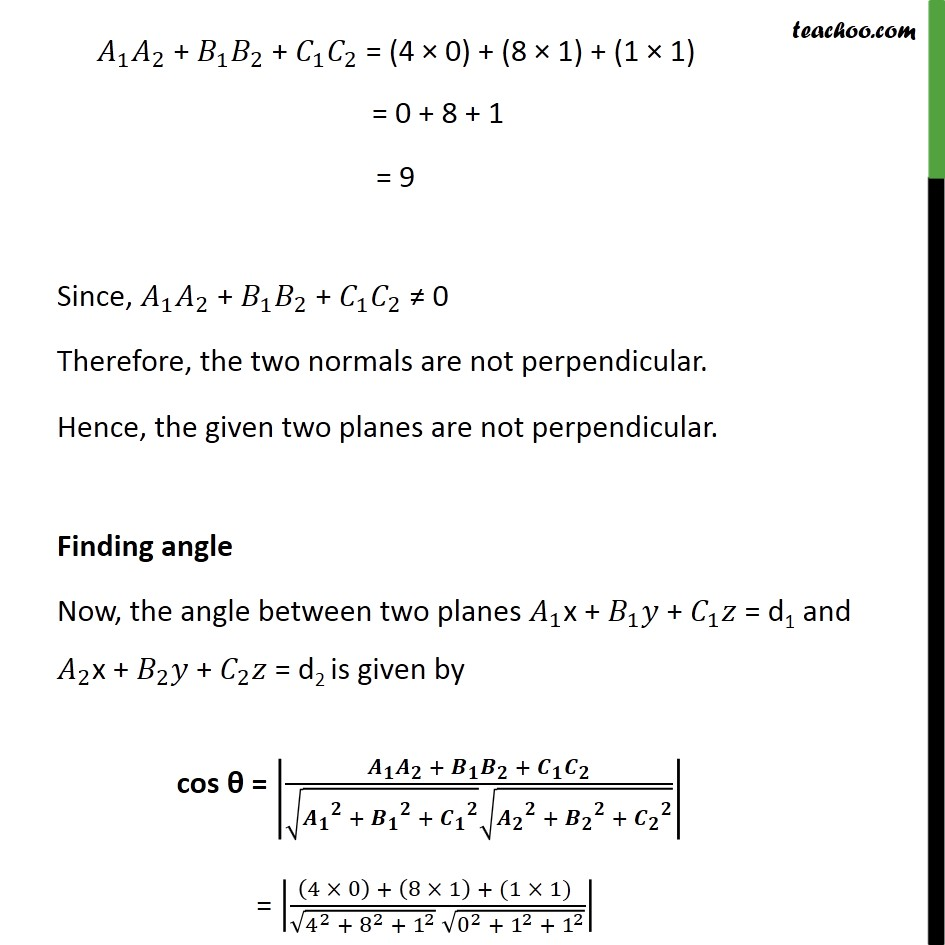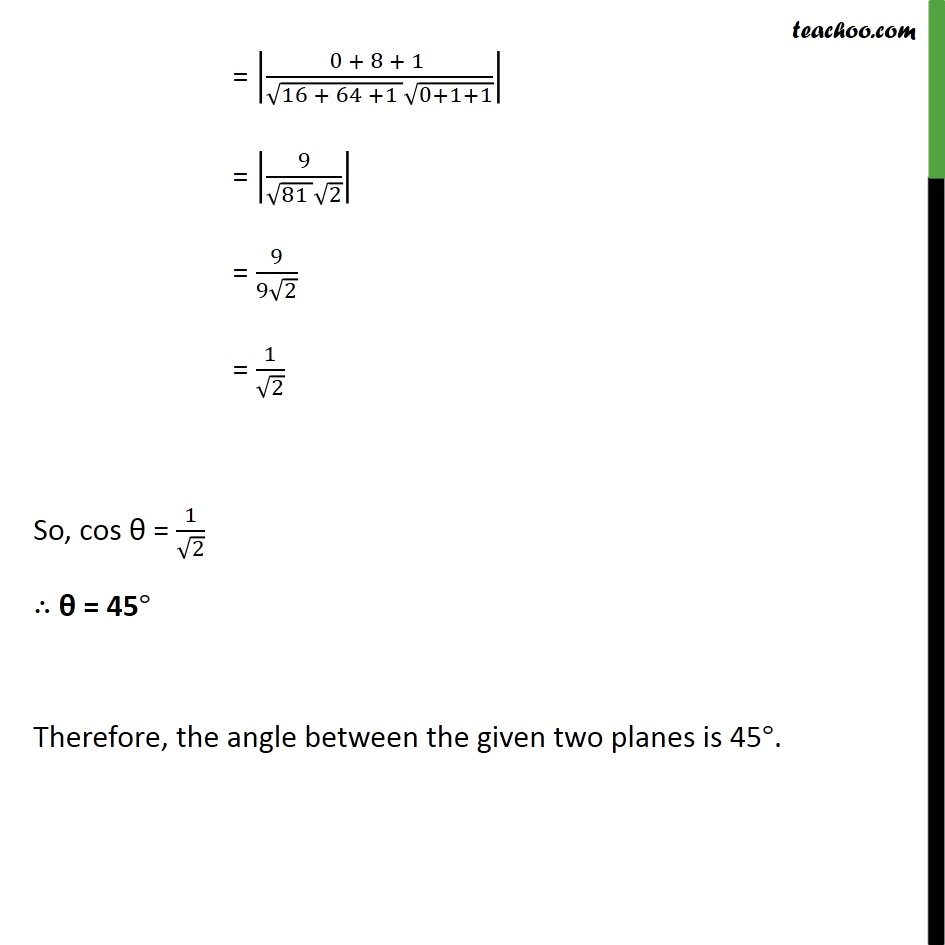1. Chapter 11 Class 12 Three Dimensional Geometry
2. Serial order wise
3. Ex 11.3

Transcript

Ex 11.3, 13 In the following cases, determine whether the given planes are parallel or perpendicular, and in case they are neither, find the angles between them. (a) 7x + 5y + 6z + 30 = 0 and 3x y 10z + 4 = 0 Given, the two planes are Check parallel Two lines with direction ratios 1 , 1 , 1 and 2 , 2 , 2 are parallel if = = So, 1 2 = 7 3 = 7 3 , 1 2 = 5 1 = 5 , 1 2 = 6 10 = 3 5 Since 1 2 1 2 1 2 So, the two normal are not parallel. Given two planes are not parallel. Check perpendicular Two lines with direction ratios 1 , 1 , 1 and 2 , 2 , 2 are perpendicular if + + = 0 1 2 + 1 2 + 1 2 = ( 7 3) + ( 5 1) + ( 6 10) = 21 + ( 5) + ( 60) = 44 Since, 1 2 + 1 2 + 1 2 0 Therefore, the two normals are not perpendicular. Hence, the given two planes are not perpendicular. Finding angle Now, the angle between two planes 1 x + 1 + 1 = d1 and 2 x + 2 + 2 = d2 is given by cos = + + + + + + = 7 3 + 5 1 + ( 6 10) 7 2 + 5 2 + 6 2 3 2 + 1 2 + 10 2 = 21 5 60 49 + 25 + 36 9 + 1 + 100 = 44 110 110 = 44 110 = 2 5 = 2 5 Hence, cos = 2 5 = Hence, angle between two planes is cos 1 2 5 Ex 11.3, 13 In the following cases, determine whether the given planes are parallel or perpendicular, and in case they are neither, find the angles between them. (b) 2x + y + 3z 2 = 0 and x 2y + 5 = 0 Given, the two planes are Check parallel Two lines with direction ratios 1 , 1 , 1 and 2 , 2 , 2 are parallel if = = So, 1 2 = 2 1 = 2, 1 2 = 1 2 , 1 2 = 3 0 Since, direction ratios are not proportional, the two normal are not parallel. Given two planes are not parallel. Check perpendicular Two lines with direction ratios 1 , 1 , 1 and 2 , 2 , 2 are perpendicular if + + = 0 Now, 1 2 + 1 2 + 1 2 = (2 1) + (1 2) + (3 0) = 2 + 2 + 0 = 0 Since 1 2 + 1 2 + 1 2 = 0 The two normals are perpendicular. Since normals are perpendicular, planes are perpendicular. Ex 11.3, 13 In the following cases, determine whether the given planes are parallel or perpendicular, and in case they are neither, find the angles between them. (c) 2x 2y + 4z + 5 = 0 and 3x 3y + 6z 1 = 0 Given, the two planes are Check parallel Two lines with direction ratios 1 , 1 , 1 and 2 , 2 , 2 are parallel if = = Here, 1 2 = 2 3 = 2, 1 2 = 2 3 = 2 3 , 1 2 = 4 6 = 2 3 Since, 1 2 = 1 2 = 1 2 = 2 3 Therefore, the two normals are parallel Since normals are parallel, the two planes are parallel. Ex 11.3, 13 In the following cases, determine whether the given planes are parallel or perpendicular, and in case they are neither, find the angles between them. (d) 2x y + 3z 1 = 0 and 2x y + 3z + 3 = 0 Given, the two planes are Check parallel Two lines with direction ratios 1 , 1 , 1 and 2 , 2 , 2 are parallel if = = Here, 1 2 = 2 2 = 1, 1 2 = 1 1 = 1, 1 2 = 3 3 = 1 Since 1 2 = 1 2 = 1 2 = 1, Therefore, the two normals are parallel Since normals are parallel, the two planes are parallel. Ex 11.3, 13 In the following cases, determine whether the given planes are parallel or perpendicular, and in case they are neither, find the angles between them. (e) 4x + 8y + z 8 = 0 and y + z 4 = 0 Given the two planes are Check parallel Two lines with direction ratios 1 , 1 , 1 and 2 , 2 , 2 are parallel if = = So, 1 2 = 4 0 = 1 , 1 2 = 8 1 = 8 , 1 2 = 1 1 = 1 Since 1 2 1 2 1 2 So, the two normal are not parallel. Given two planes are not parallel. Check perpendicular Two lines with direction ratios 1 , 1 , 1 and 2 , 2 , 2 are perpendicular if + + = 0 1 2 + 1 2 + 1 2 = (4 0) + (8 1) + (1 1) = 0 + 8 + 1 = 9 Since, 1 2 + 1 2 + 1 2 0 Therefore, the two normals are not perpendicular. Hence, the given two planes are not perpendicular. Finding angle Now, the angle between two planes 1 x + 1 + 1 = d1 and 2 x + 2 + 2 = d2 is given by cos = + + + + + + = 4 0 + 8 1 + (1 1) 4 2 + 8 2 + 1 2 0 2 + 1 2 + 1 2 = 0 + 8 + 1 16 + 64 +1 0+1+1 = 9 81 2 = 9 9 2 = 1 2 So, cos = 1 2 = 45 Therefore, the angle between the given two planes is 45 .

Ex 11.3